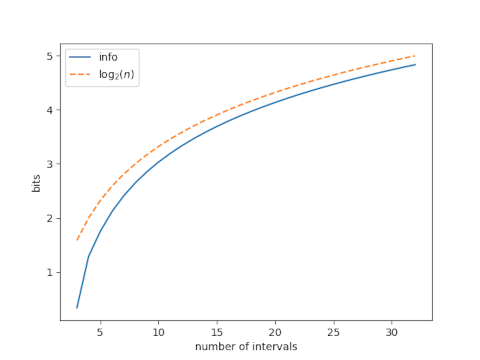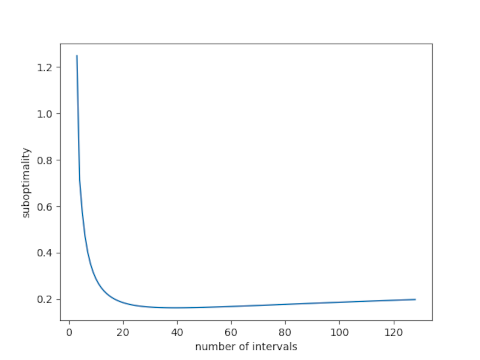# Information in a discretized normal

Here’s a problem that came up in my work today.

Suppose you have a normal random variable X and you observe X in n discrete bins. The first bin is the left α tail, the last bin is the right α tail, and the range between the two tails is divided evenly into n-2 intervals. How many bits of information are in such an observation?

For example, assume adult male heights are normally distributed with mean 70 inches and standard deviation 3 inches. How much information is there in an observation of height, rounded down to the nearest inch, if we put all heights less than 64 inches in a bin and all heights greater than or equal to 76 inches in a bin?

Here α = 0.025 because that’s the probability that a normal random variable is more than 2 standard deviations below its mean or more than 2 standard deviations above its mean.

We have n = 15 because we have intervals (-∞, 64), [64, 65), [65, 66), … [75, 76), and [76, ∞).

We know that with n bins we have at most log2n bits of information. That would correspond to n bins of equal probability. In other words, we’d get the maximum information if we spaced our bins evenly in terms of percentiles rather in terms of inches.

So how does the information content vary as a function of the number of bins, and how close is it to the maximum information for n bins?

Here’s a plot, with α = 0.025.The suboptimality of our bins, i.e. the difference between log2n and the information we get from n bins, drops quickly at first as n increases, then appears to plateau.

Next lets look at just the suboptimality, and increase our range of n.This shows that the suboptimality does not approach an asymptote but instead starts to increase. The minimum at n = 36 is 0.16 bits.

## One thought on “Information in a discretized normal”

1. Douglas

Naively I would have thought the minimum would be 40 bins (or 38 not counting first and last), as that would give 2.5% in each bin (if placed optimally).
I guess the minimum of 36 comes from the non-optimal placement of the bins?